Alakh Sethi — March 4, 2020

## Introduction

The Transform function in Pandas (Python) can be slightly difficult to understand, especially if you’re coming from an Excel background. Honestly, most data scientists don’t use it right off the bat in their learning journey.

But Pandas’ transform function is actually quite a handy tool to have as a data scientist! It is a powerful function that you can lean on for feature engineering in Python.I personally started using this when I was looking to perform feature engineering in a hackathon – and I was pleasantly surprised by how quickly the Transform function worked. I strongly feel you will benefit from knowing and using the Transform function and hence I decided to write about it in this article.

To learn the basics of Python and Pandas for data science, check out these popular courses:

1. What is the Transform Function in Python?
2. Why is the Transform Function Important?
3. Apply vs. Transform Function in Python

## 1. What is the Transform Function in Python?

Python’s Transform function returns a self-produced dataframe with transformed values after applying the function specified in its parameter. This dataframe has the same length as the passed dataframe.

That was a lot to take in so let me break it down using an example.

Let’s say we want to multiply 10 to each element in a dataframe:

The original dataframe looks like this: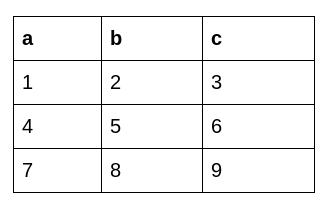This is the dataframe we get after applying Python’s Transform function: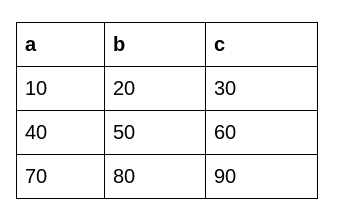## 2. Why is Python’s Transform Function Important?

Transform comes in handy during feature extraction. As the name suggests, we extract new features from existing ones. Let’s understand the importance of the transform function with the help of an example.

Here, we have a dataset about a department store: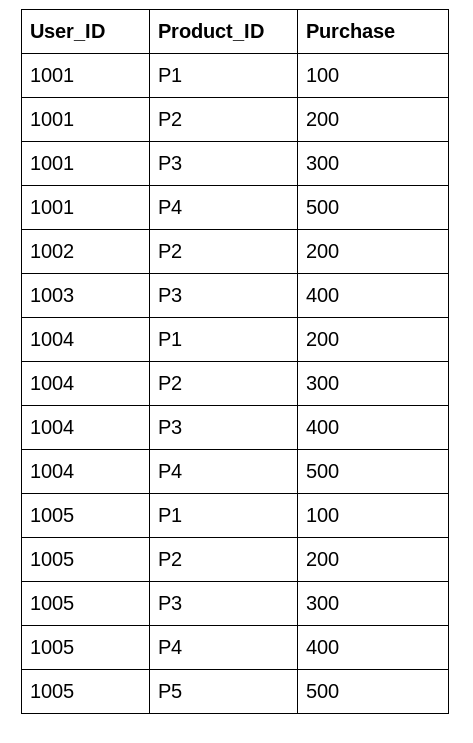We can see that each user has bought multiple products with different purchase amounts. We would like to know what is the mean purchase amount of each user. This helps us in creating a new feature for the model to understand the relationship better.

This is the desired output: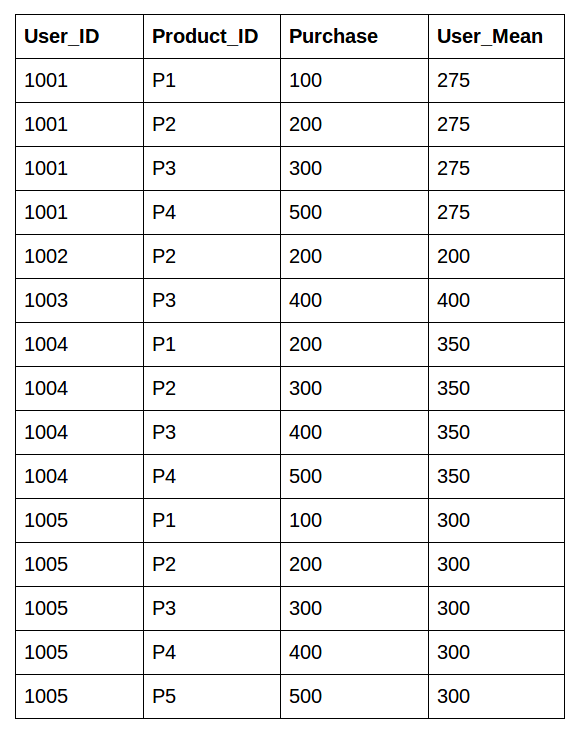There are multiple approaches to do this:

1. Using Groupby followed by merge()
2. Transform function approach

### Approach 1: Using Groupby followed by merge():

The first approach is using groupby to aggregate the data then merge this data back into the original dataframe using the merge() function. Let’s do it!

#### Step2: Use groupby to calculate the aggregate

Here is a pictorial representation of how groupby puts together the mean of each user: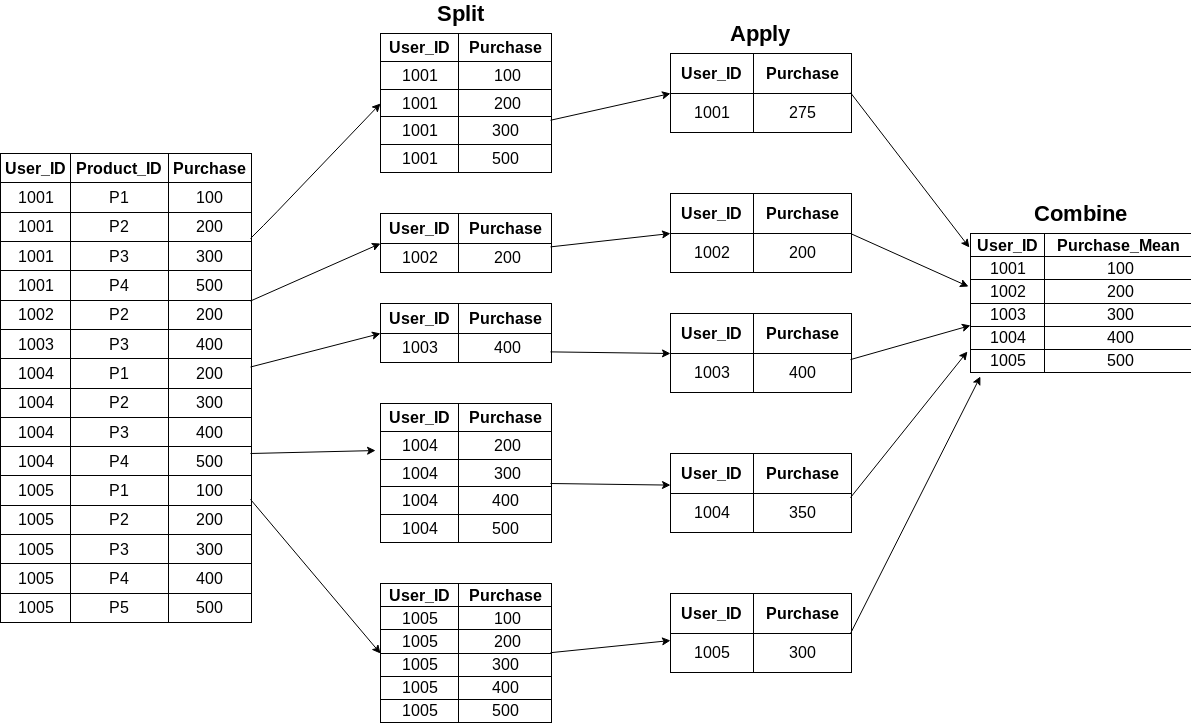#### Step3: Using merge() function to recombine

Now the tough part. How do we combine this data back to the original dataframe? We’ll be using the merge() function for this task. You can read more about joins and merges in Python using Pandas here and here, respectively.

Our original dataframe looks like this:This certainly does our work. But it is a multistep process and requires extra code to get the data in the form we require. This multistep process can be resource-consuming in hackathons where time is a major constraint.

We can solve this effectively using the transform function in Pandas.

### Approach 2: Using Python’s Transform Function

This an important function for creating features. Trust me, it can be game-changer!

The transform function retains the same number of items as the original dataset after performing the transformation. We’ll be leaning on a super-easy one-line step using groupby followed by a transform:

The pictorial representation is as follows: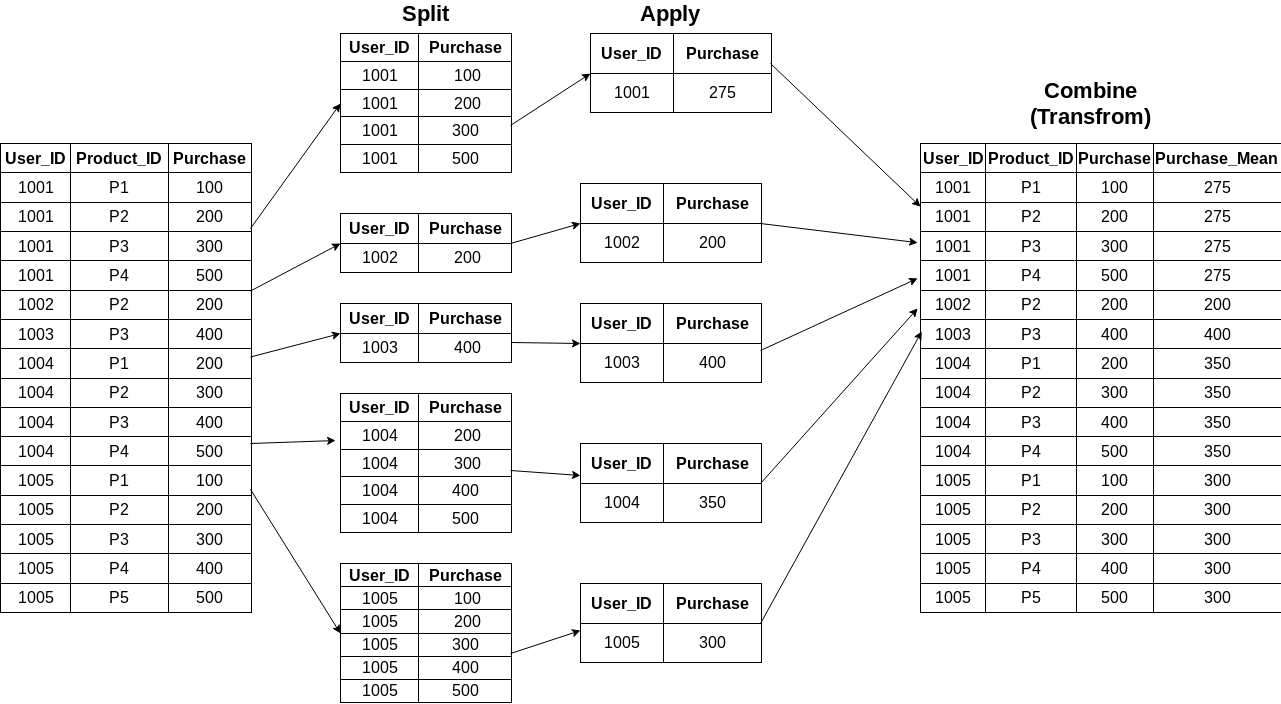Couldn’t be simpler, right? The original dataframe looks similar to the above one in the last step.

The time taken by the transform function to perform the above operation is comparatively less over a large dataframe. That’s a sigificant advantage as comapred to the first approach we used.

Let me demonstrate the Transform function using Pandas in Python.

Suppose we create a random dataset of 1,000,000 rows and 3 columns. Now we calculate the mean of one column based on groupby (similar to mean of all purchases based on groupby user_id).

#### Step 4: Use the transform function

This clearly shows the transform function is much faster than the previous approach. Well done!

## 3. Difference Between Apply And Transform Function in Python

Now, let’s say we want to create a new column based on the values of another column. This is the dataframe we’re working with:With the apply function:

This is what the output looks like using the Apply function: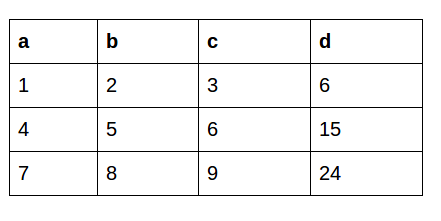The apply function sends a whole copy of the dataframe to work upon so we can manipulate all the rows or columns simultaneously.

With the Transform function:

This feature is not possible in the Transform function. This just manipulates a single row or column based on axis value and doesn’t manipulate a whole dataframe. So, we can use either Apply or the Transform function depending on the requirement.

## End Notes

The Transform function is super useful when I’m quickly looking to manipulate rows or columns. As I mentioned earlier this comes in especially handy in hackathons when time is of the essence.

If you come across any more such Pandas functions, do comment and I’ll be happy to learn and share!

Start participating in competitions to showcase your skills. This is a great starting point: Black Friday Sales Project.

You can register on the DataHack platform and participate in cool competitions and compete with the best data science experts!